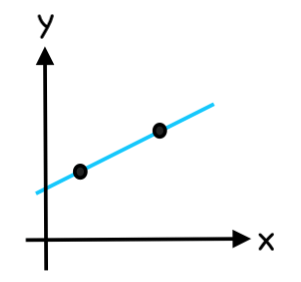# A short explanation

Calculus is divided into two parts – differentiation and integration.

Differential Calculus answers the question “How do you find the instantaneous rate of change?”

Integral Calculus answers the questions “How do you find the area under a curve?”

Mortensen Math focuses on differentiation and finding the derivative of the linear function f(x)=mx+b using the difference quotient.Various types of change can be described by a derivative. Derivatives allow us to find out how fast something is changing or its rate of change. The derivative at a chosen point on a line or curve describes the rate of change of the function near that point. The process of finding a derivative is called differentiation.

Finding the rate of change at a given point on a straight line is simple because it is the same at each point – it’s constant.The rate of change or slope stays the same at every point on a line.

Finding the rate of change at a point on a curve is more difficult because the slope is different at every point – it’s changing.The rate of change or slope changes at every point on a curve.

When we draw a line connecting two points on a curve we call the line a secant line. The slope of the secant line is the average rate of change of the curve between the two points.

As the distance between the two points approach zero the secant line approaches a tangent line.